# Chapter 8 Chemical Equations Reactions 8 1 Describing

• Slides: 50Chapter 8 -Chemical Equations & Reactions 8. 1 -Describing Chemical Reactions 8. 2 -Types of Chemical Reactions 8. 3 -Activity Series of the Elements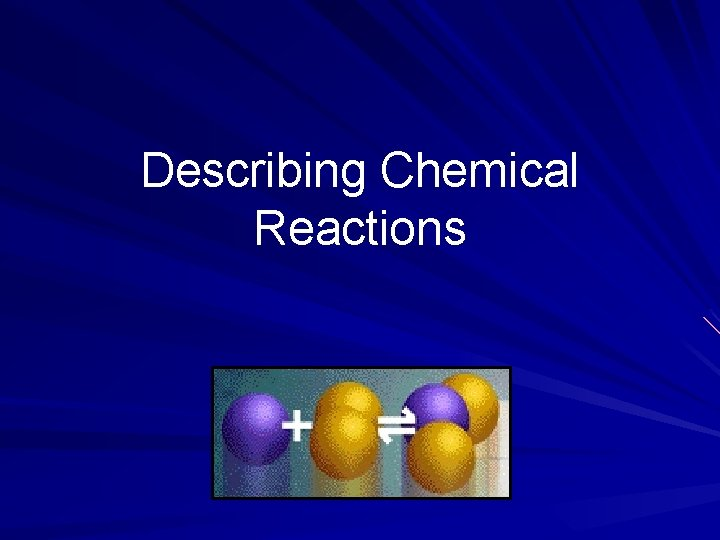Describing Chemical Reactions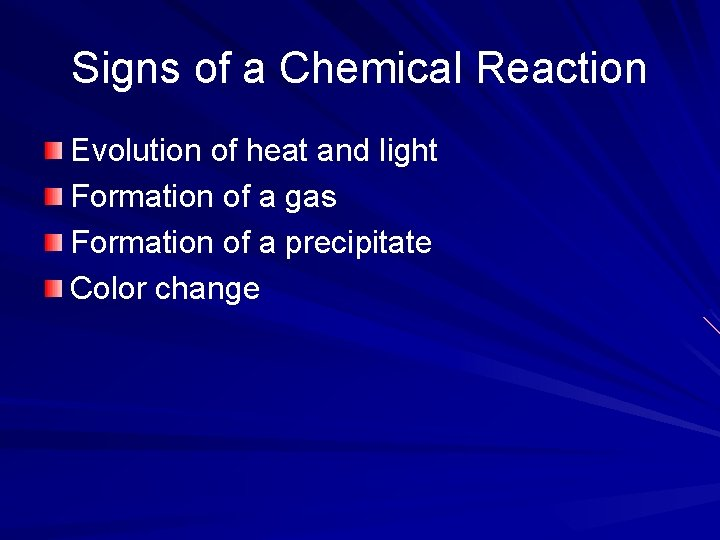Signs of a Chemical Reaction Evolution of heat and light Formation of a gas Formation of a precipitate Color change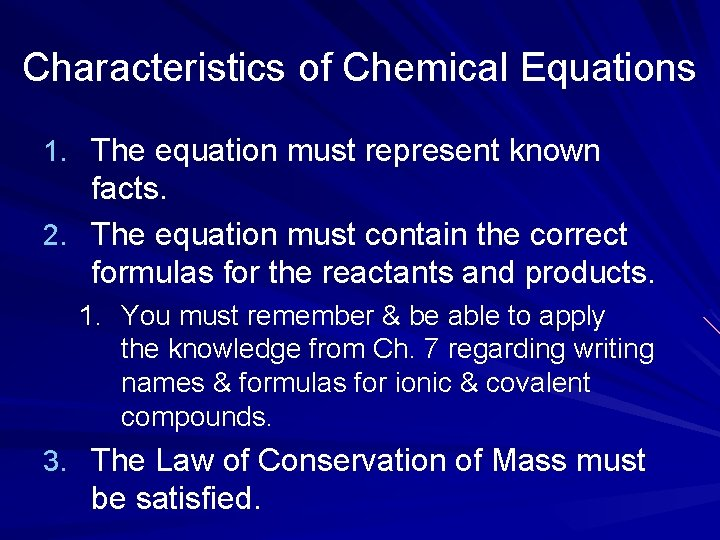Characteristics of Chemical Equations 1. The equation must represent known facts. 2. The equation must contain the correct formulas for the reactants and products. 1. You must remember & be able to apply the knowledge from Ch. 7 regarding writing names & formulas for ionic & covalent compounds. 3. The Law of Conservation of Mass must be satisfied.Law of Conservation of Mass is neither created nor destroyed in a chemical reaction – Total mass stays the same – Atoms can only rearrange 4 H 36 g 2 O 4 g 32 g 4 H 2 OChemical Equations A+B C+D REACTANTS PRODUCTS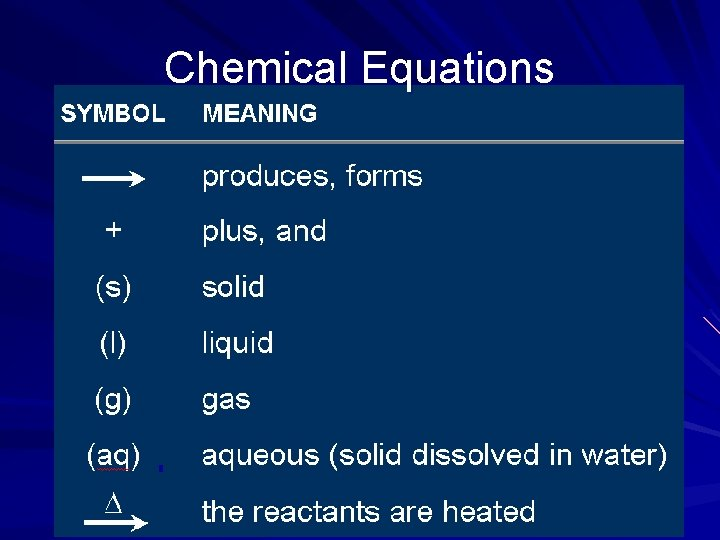Chemical EquationsWord vs. Formula/Chemical Eqns. Word equations are equations in which the reactants & products are represented by words. – Methane + oxygen carbon dioxide + water Formula equations are equations in which the reactants & products are represented by their symbols or formulas. – CH 4(g) + O 2(g) CO 2(g) + H 2 O(g)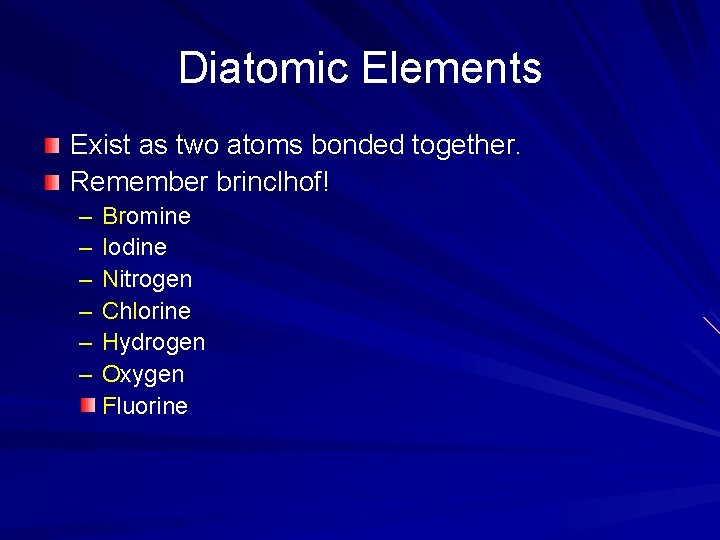Diatomic Elements Exist as two atoms bonded together. Remember brinclhof! – – – Bromine Iodine Nitrogen Chlorine Hydrogen Oxygen Fluorine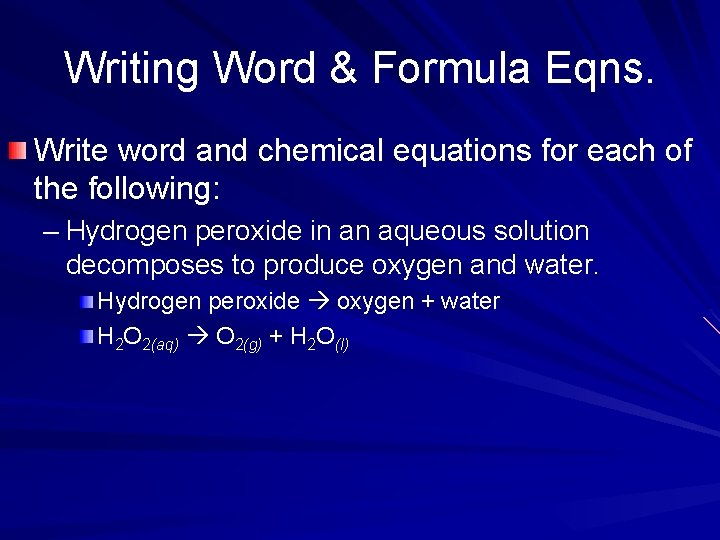Writing Word & Formula Eqns. Write word and chemical equations for each of the following: – Hydrogen peroxide in an aqueous solution decomposes to produce oxygen and water. Hydrogen peroxide oxygen + water H 2 O 2(aq) O 2(g) + H 2 O(l)Writing Word & Formula Eqns. – Solid copper metal reacts with aqueous silver nitrate to produce solid silver metal and aqueous copper(II) nitrate. Copper + silver nitrate silver + copper(II) nitrate Cu(s) + Ag. NO 3(aq) Ag(s) + Cu(NO 3)2(aq) – Solid zinc metal reacts with aqueous copper(II) sulfate to produce solid copper metal and aqueous zinc sulfate. Zinc + copper(II) sulfate copper + zinc sulfate Zn(s) + Cu. SO 4(aq) Cu(s) + Zn. SO 4(aq)Balancing Equations In a chemical equation, the law of conservation of mass is satisfied by “balancing” the number of atoms of each element in the reactants and products.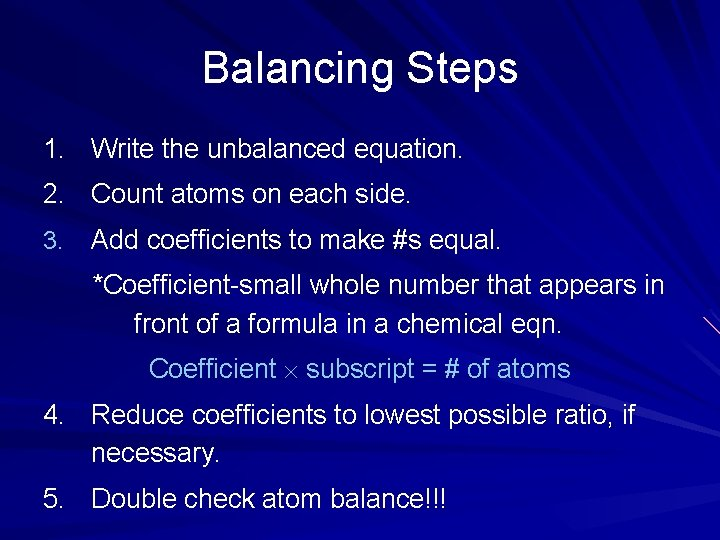Balancing Steps 1. Write the unbalanced equation. 2. Count atoms on each side. 3. Add coefficients to make #s equal. *Coefficient-small whole number that appears in front of a formula in a chemical eqn. Coefficient subscript = # of atoms 4. Reduce coefficients to lowest possible ratio, if necessary. 5. Double check atom balance!!!Helpful Tips Balance one element at a time. Update ALL atom counts after adding a coefficient. If an element appears more than once per side, balance it last. Balance polyatomic ions as single units. – “ 1 SO 4” instead of “ 1 S” and “ 4 O”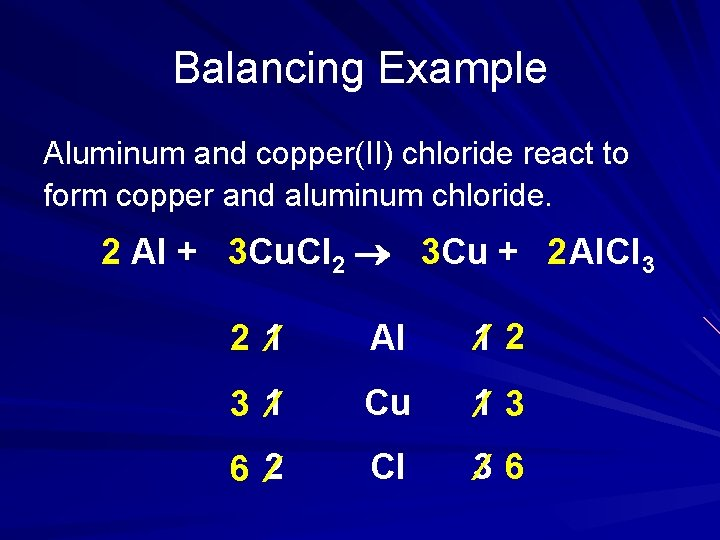Balancing Example Aluminum and copper(II) chloride react to form copper and aluminum chloride. 2 Al + 3 Cu. Cl 2 3 Cu + 2 Al. Cl 3 2 1 Al 1 2 3 1 Cu 1 3 6 2 Cl 3 6Balancing Examples H 2 O 2(aq) O 2(g) + H 2 O(l) – 2 H 2 O 2(aq) O 2(g) + 2 H 2 O(l) Cu(s) + Ag. NO 3(aq) Ag(s) + Cu(NO 3)2(aq) Cu(s) + 2 Ag. NO 3(aq) 2 Ag(s) + Cu(NO 3)2(aq) Zn(s) + Cu. SO 4(aq) Cu(s) + Zn. SO 4(aq)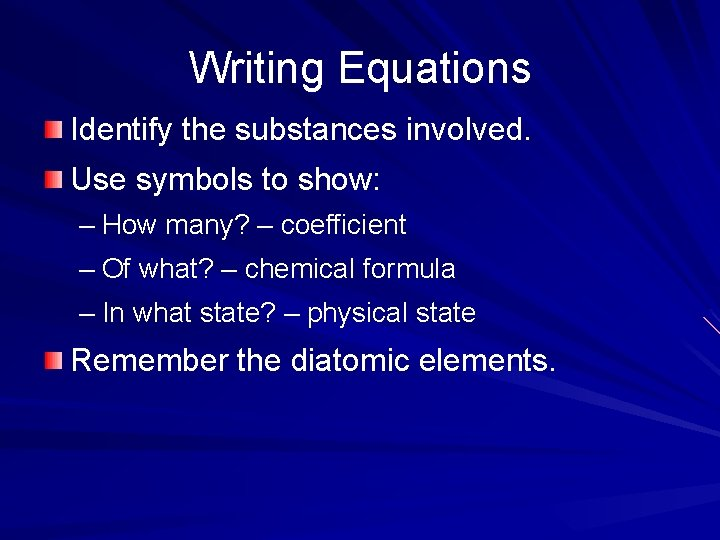Writing Equations Identify the substances involved. Use symbols to show: – How many? – coefficient – Of what? – chemical formula – In what state? – physical state Remember the diatomic elements.Describing Equations Describing Coefficients: – individual atom = “atom” – covalent substance = “molecule” – ionic substance = “unit” 3 CO 2 3 molecules of carbon dioxide 2 Mg 2 atoms of magnesium 4 Mg. O 4 units of magnesium oxide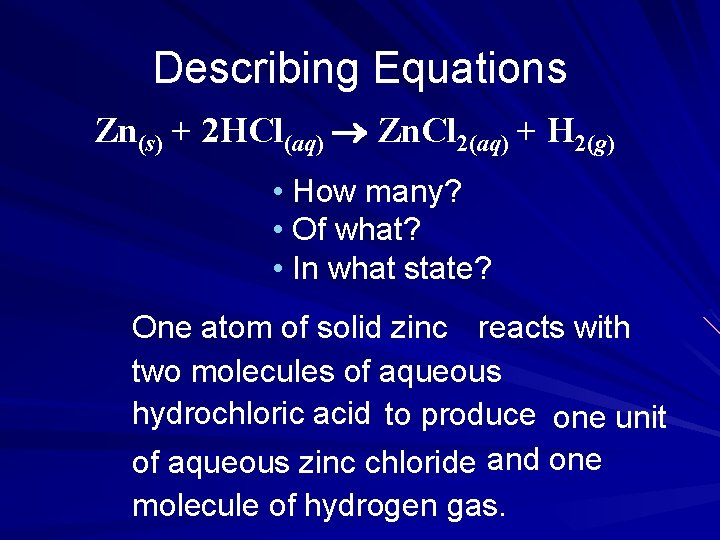Describing Equations Zn(s) + 2 HCl(aq) Zn. Cl 2(aq) + H 2(g) • How many? • Of what? • In what state? One atom of solid zinc reacts with two molecules of aqueous hydrochloric acid to produce one unit of aqueous zinc chloride and one molecule of hydrogen gas.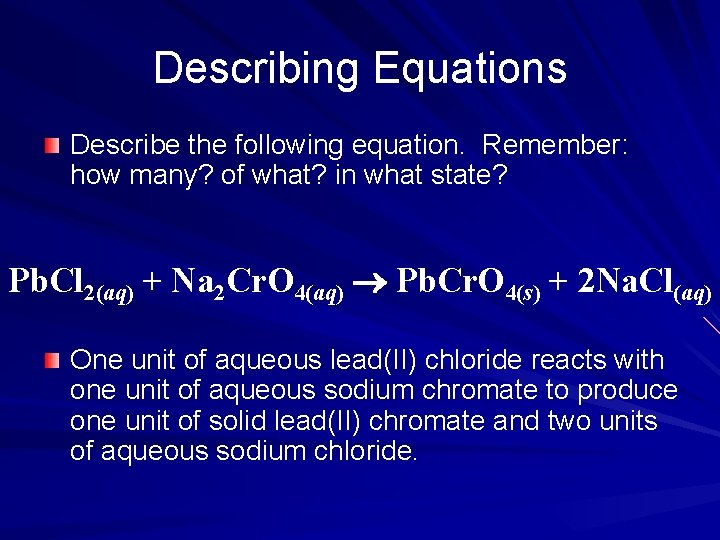Describing Equations Describe the following equation. Remember: how many? of what? in what state? Pb. Cl 2(aq) + Na 2 Cr. O 4(aq) Pb. Cr. O 4(s) + 2 Na. Cl(aq) One unit of aqueous lead(II) chloride reacts with one unit of aqueous sodium chromate to produce one unit of solid lead(II) chromate and two units of aqueous sodium chloride.Review Write balanced chemical equations for each of the following reactions: – Solid sodium combines with chlorine gas to produce solid sodium chloride. – When solid copper reacts with aqueous silver nitrate, the products are aqueous copper(II) nitrate & solid silver. – In a blast furnace, the reaction between solid iron(III) oxide & carbon monoxide gas produces solid iron & carbon dioxide gas. 2 Na(s) + Cl 2(g) 2 Na. Cl(s) Cu(s) + 2 Ag. NO 3(aq) Cu(NO 3)2(aq) + 2 Ag(s) Fe 2 O 3(s) + 3 CO(g) 2 Fe(s) + 3 CO 2(g)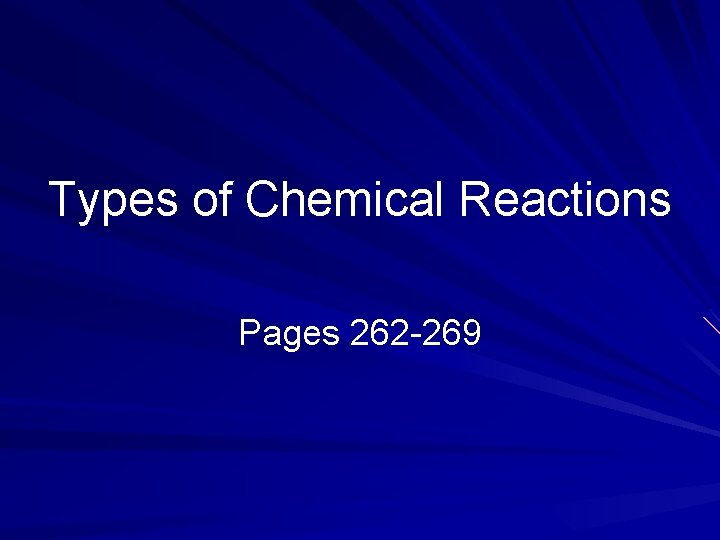Types of Chemical Reactions Pages 262 -269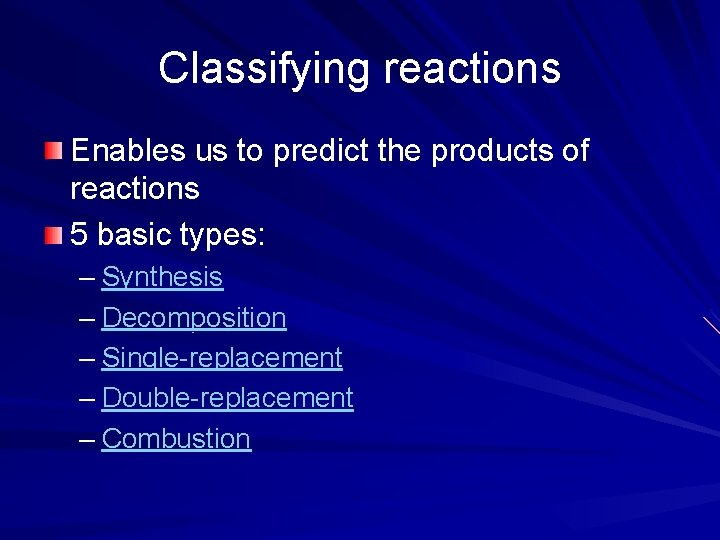Classifying reactions Enables us to predict the products of reactions 5 basic types: – Synthesis – Decomposition – Single-replacement – Double-replacement – CombustionSynthesis AKA composition reaction the combination of 2 or more substances to form a compound only one product A + B ABSynthesis H 2(g) + Cl 2(g) 2 HCl(g)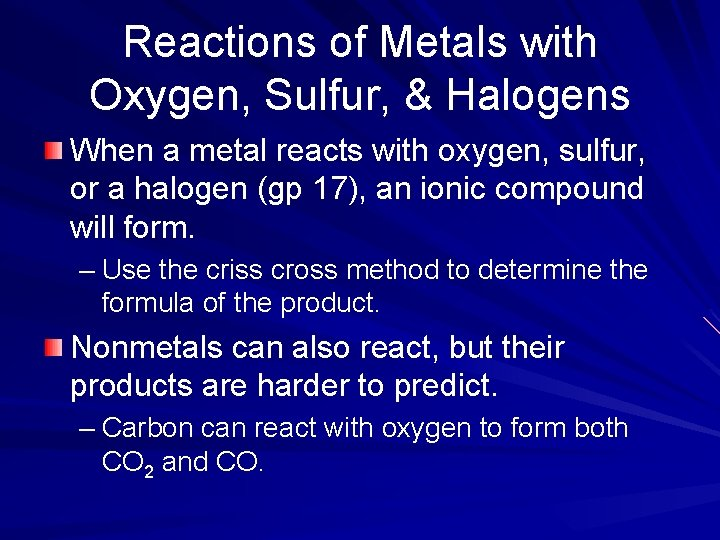Reactions of Metals with Oxygen, Sulfur, & Halogens When a metal reacts with oxygen, sulfur, or a halogen (gp 17), an ionic compound will form. – Use the criss cross method to determine the formula of the product. Nonmetals can also react, but their products are harder to predict. – Carbon can react with oxygen to form both CO 2 and CO.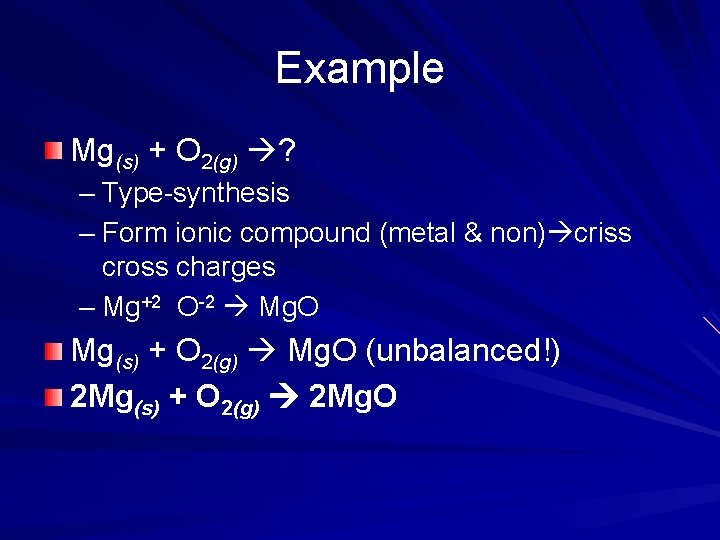Example Mg(s) + O 2(g) ? – Type-synthesis – Form ionic compound (metal & non) criss cross charges – Mg+2 O-2 Mg. O Mg(s) + O 2(g) Mg. O (unbalanced!) 2 Mg(s) + O 2(g) 2 Mg. ODecomposition a compound breaks down into 2 or more simpler substances only one reactant AB A + B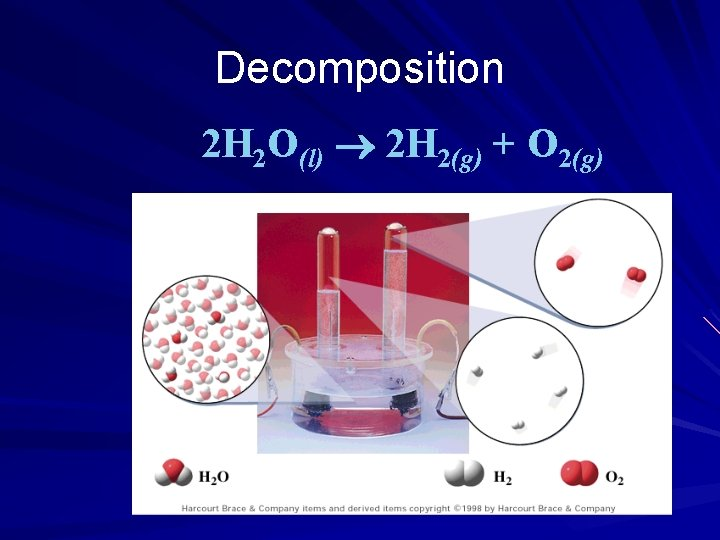Decomposition 2 H 2 O(l) 2 H 2(g) + O 2(g)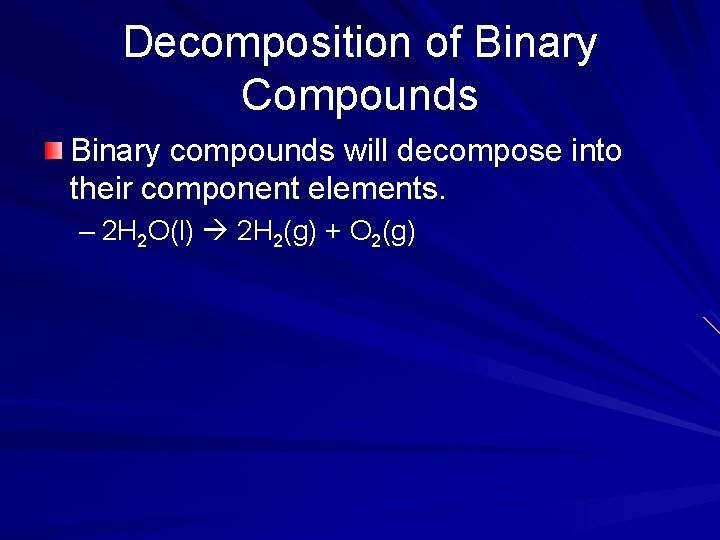Decomposition of Binary Compounds Binary compounds will decompose into their component elements. – 2 H 2 O(l) 2 H 2(g) + O 2(g)Decomposition of Metal Carbonates Ionic compounds formed from a metal cation and the carbonate anion (CO 32 -) will decompose to form a metal oxide and carbon dioxide. – Ca. CO 3(s) Ca. O(s) + CO 2(g)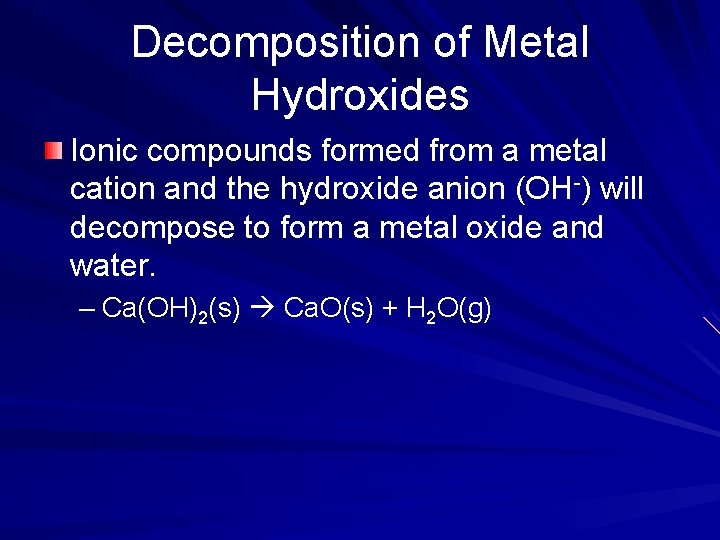Decomposition of Metal Hydroxides Ionic compounds formed from a metal cation and the hydroxide anion (OH-) will decompose to form a metal oxide and water. – Ca(OH)2(s) Ca. O(s) + H 2 O(g)Decomposition of Metal Chlorates Ionic compounds formed from a metal cation and the chlorate anion (Cl. O 3 -) will decompose to form a metal chloride and oxygen. – 2 KCl. O 3(s) 2 KCl(s) + 3 O 2(g)Decomposition of Acids Certain acids decompose to form nonmetal oxides and water. – Carbonic acid (H 2 CO 3) will decompose to form H 2 O and CO 2. – Sulfuric acid (H 2 SO 4) will decompose into water and sulfur trioxide.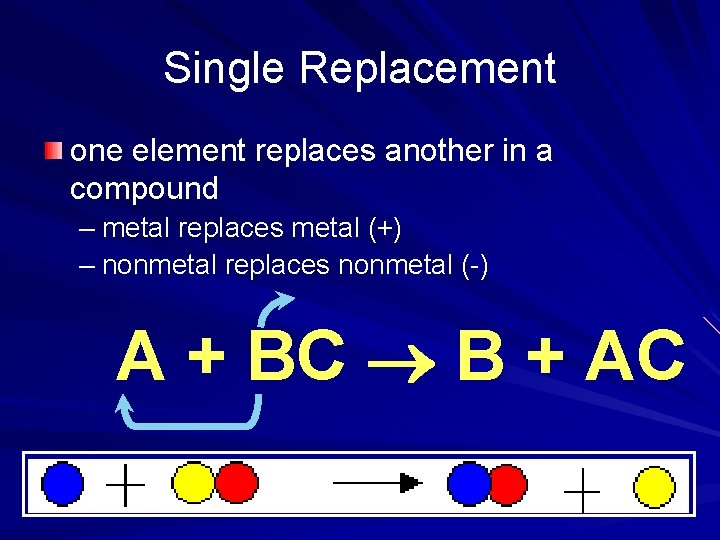Single Replacement one element replaces another in a compound – metal replaces metal (+) – nonmetal replaces nonmetal (-) A + BC B + ACSingle Replacement Cu(s) + 2 Ag. NO 3(aq) Cu(NO 3)2(aq) + 2 Ag(s)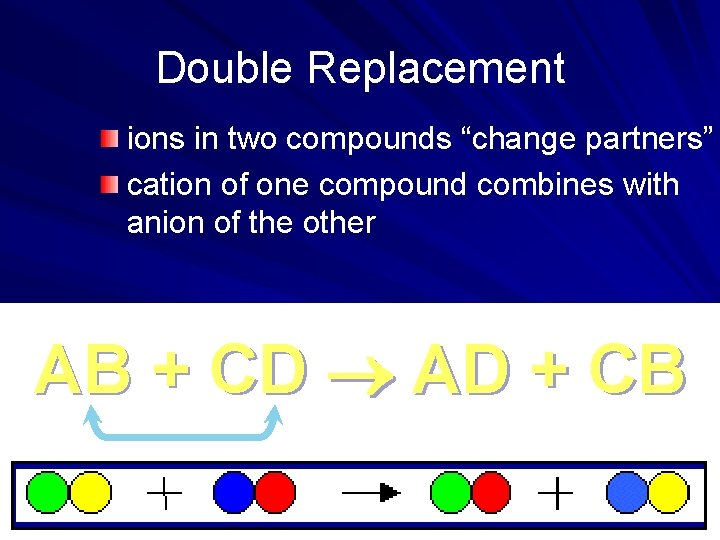Double Replacement ions in two compounds “change partners” cation of one compound combines with anion of the other AB + CD AD + CBDouble Replacement Pb(NO 3)2(aq) + K 2 Cr. O 4(aq) Pb. Cr. O 4(s) + 2 KNO 3(aq)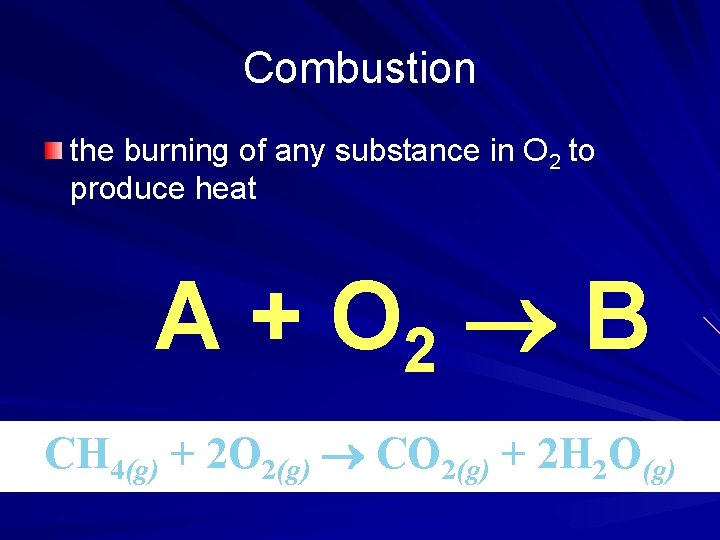Combustion the burning of any substance in O 2 to produce heat A + O 2 B CH 4(g) + 2 O 2(g) CO 2(g) + 2 H 2 O(g)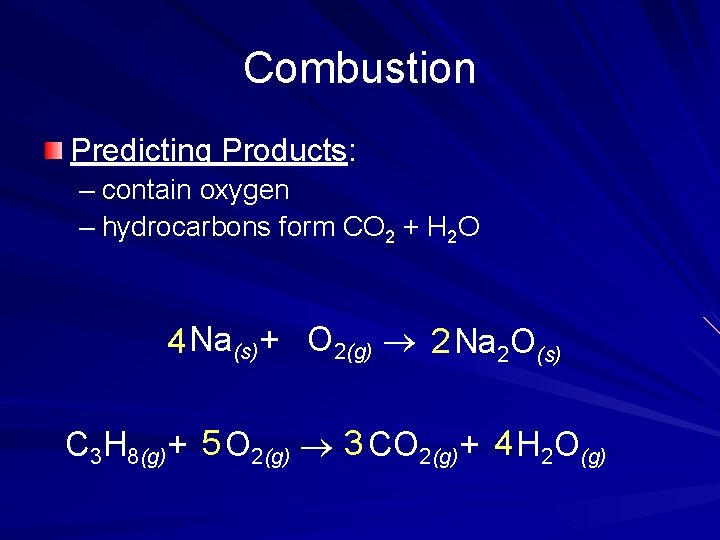Combustion Predicting Products: – contain oxygen – hydrocarbons form CO 2 + H 2 O 4 Na(s)+ O 2(g) 2 Na 2 O(s) C 3 H 8(g)+ 5 O 2(g) 3 CO 2(g)+ 4 H 2 O(g)Classify each rxn: N 2(g) + 3 H 2(g) 2 NH 3(g) 2 C 6 H 14(l) + 19 O 2(g) 12 CO 2(g) + 14 H 2 O(g) Ag. NO 3(aq) + Na. Cl(aq) Ag. Cl(s) + Na. NO 3(aq) Synthesis Combustion Double replacement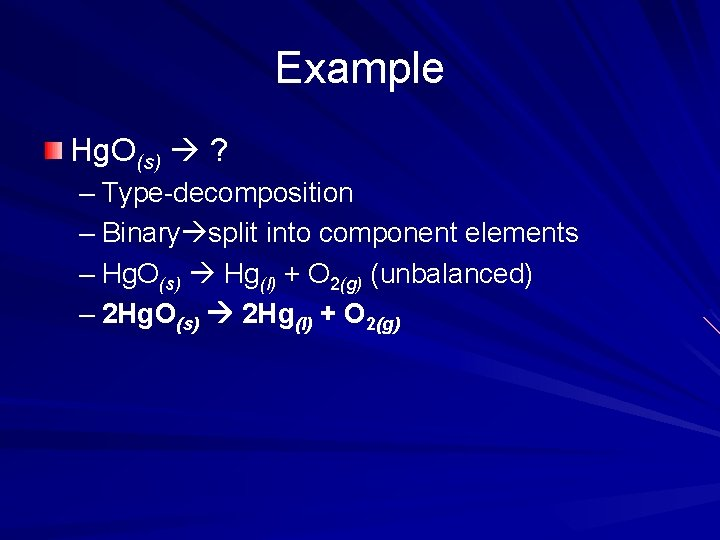Example Hg. O(s) ? – Type-decomposition – Binary split into component elements – Hg. O(s) Hg(l) + O 2(g) (unbalanced) – 2 Hg. O(s) 2 Hg(l) + O 2(g)Predicting products: double replacement rxns AB + CD AD + CB For this type of rxn to occur, at least one of the products must be insoluble in water (precipitate or be a solid) Check solubility rules/table – Rules pg. R 64 – Table B-12Example Ag. NO 3(aq) + Na. Cl(aq) ? – Type-double replacement – Expected products: Ag. Cl and Na. NO 3 – Check the solubility of these compounds Insoluble, soluble Rxn will occur b/c a product is insoluble Ag. NO 3(aq) + Na. Cl(aq) Ag. Cl(s) + Na. NO 3(aq)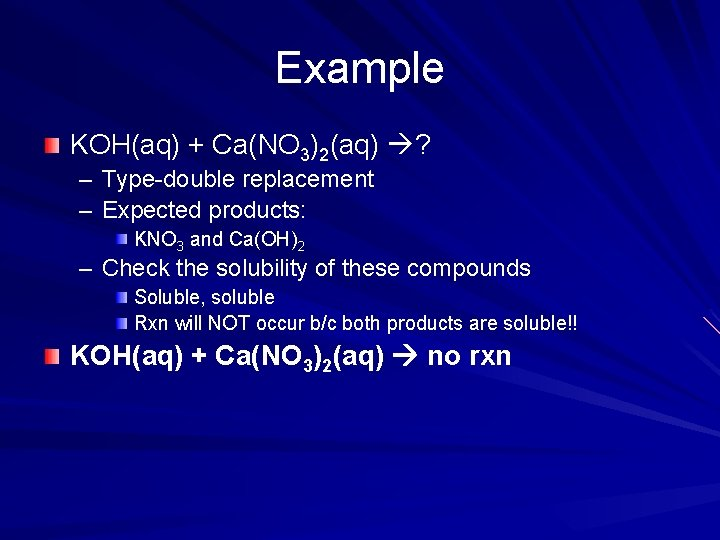Example KOH(aq) + Ca(NO 3)2(aq) ? – Type-double replacement – Expected products: KNO 3 and Ca(OH)2 – Check the solubility of these compounds Soluble, soluble Rxn will NOT occur b/c both products are soluble!! KOH(aq) + Ca(NO 3)2(aq) no rxn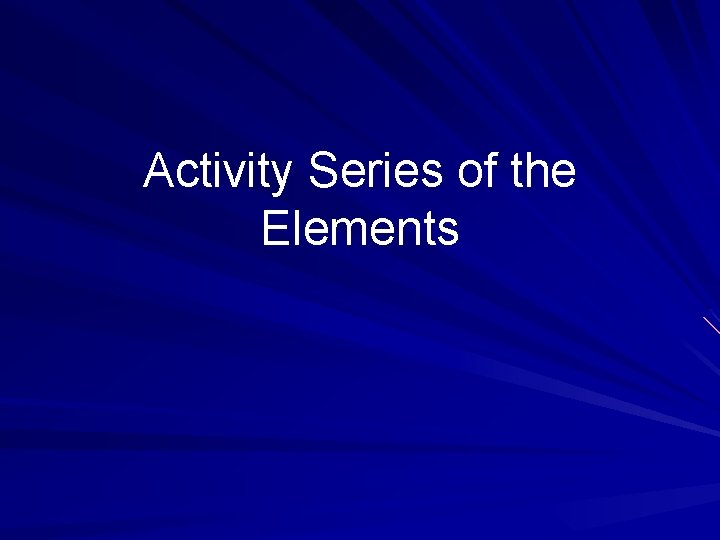Activity Series of the ElementsPredicting products: single replacement rxns A + BC B + AC To determine if A will replace B in compound BC, use the activity series Activity series-list of elements organized according to the ease with which the elements undergo certain chemical reactions. An element can replace any element below it in the series. Pg. 266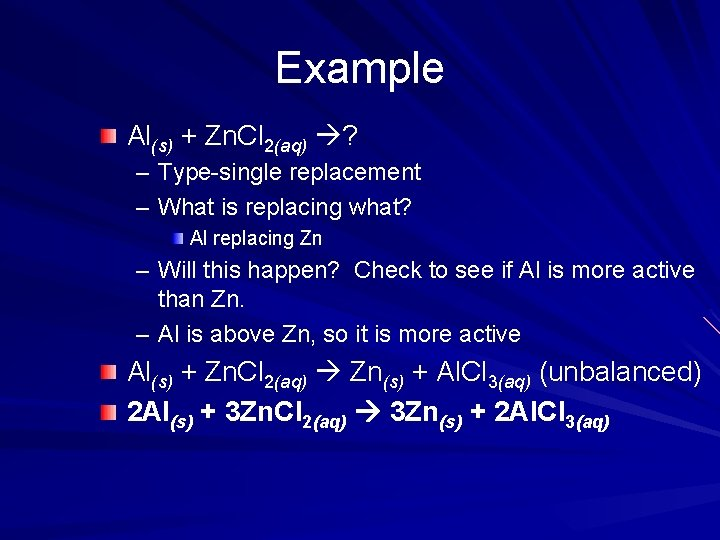Example Al(s) + Zn. Cl 2(aq) ? – Type-single replacement – What is replacing what? Al replacing Zn – Will this happen? Check to see if Al is more active than Zn. – Al is above Zn, so it is more active Al(s) + Zn. Cl 2(aq) Zn(s) + Al. Cl 3(aq) (unbalanced) 2 Al(s) + 3 Zn. Cl 2(aq) 3 Zn(s) + 2 Al. Cl 3(aq)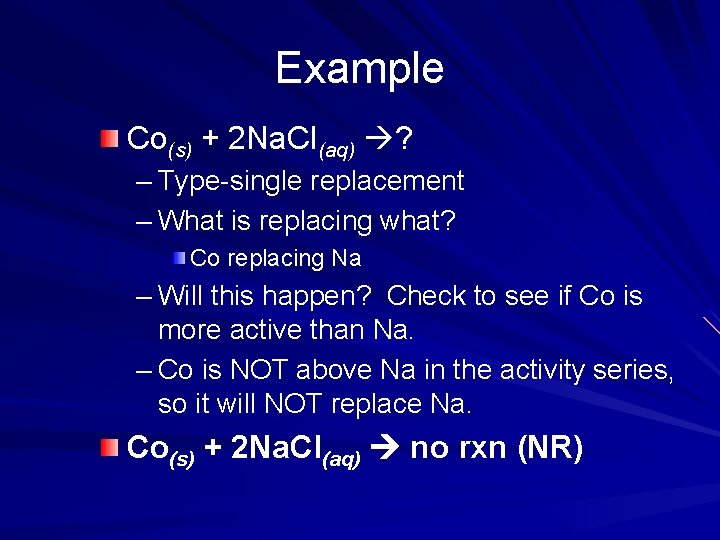Example Co(s) + 2 Na. Cl(aq) ? – Type-single replacement – What is replacing what? Co replacing Na – Will this happen? Check to see if Co is more active than Na. – Co is NOT above Na in the activity series, so it will NOT replace Na. Co(s) + 2 Na. Cl(aq) no rxn (NR)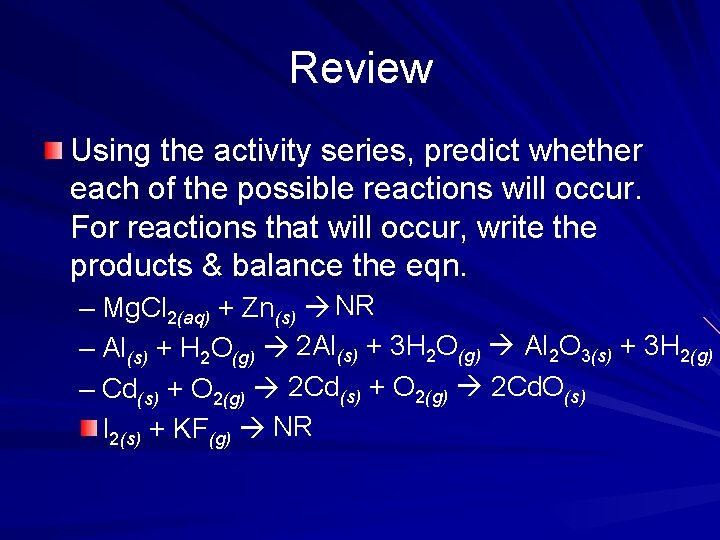Review Using the activity series, predict whether each of the possible reactions will occur. For reactions that will occur, write the products & balance the eqn. – Mg. Cl 2(aq) + Zn(s) NR – Al(s) + H 2 O(g) 2 Al(s) + 3 H 2 O(g) Al 2 O 3(s) + 3 H 2(g) – Cd(s) + O 2(g) 2 Cd. O(s) I 2(s) + KF(g) NR